SBI CLERK Quantitative Aptitude Quiz

Here, we are providing SBI CLERK Mains Study plan as there is left not enough time to deal in details. The questions asked in the quantitative aptitude section are calculative and very time-consuming. But once dealt with proper strategy, speed, and accuracy, this section can get you the maximum marks in the examination. Following is the Quantitative Aptitude quiz to help you practice with the best of latest pattern questions

Directions (1-4): In the given pie chart, in state Bank of India there are two types of accounts Saving account and Current account which can be opened by an Indian.
These pie charts show the percentage wise breakup of these accounts spend in a given year. There are 4 quarters in a year and graph shown the informQ1. If we include the 4th quarter in the given year, percentage of Current accounts opened in 2nd quarter will become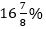of the total Current accounts opened during the whole year. Then what is the number of Current accounts opened in 4th quarter?
450
300
350
250
400

Q2. Total number of Saving accounts opened in the 4th quarter aremore than the Saving accounts opened in 3rd quarter, then find the average number of Saving accounts opened during whole year.
325
200
350
250
300

Q3. If the total number of Saving accounts opened in the whole year are 50% more than the Current account opened in the whole year, then find the ratio of the Saving accounts opened in 4th quarter to Current accounts opened in 4th quarter?
2 : 3
2 : 5
3 : 2
Can’t be determined
3: 5

Q4. If the Saving accounts opened in 4th quarter is 240 more than the Saving accounts opened in 2nd quarter, then Saving accounts opened in 4th quarter is what percent of the total Saving accounts opened in the whole year?
37.5%
39.5%
35.5%
36%
32.5%

Directions (5-10): What will come at the place of question mark in the following questions? (You are not expected to find exact value)

Q5. 16.978 + 27.007 + 36.984 - 12.969 - 9.003 = ?

72
42
60
51
65

Q6. 499.97÷4.95+(5.99)³-207.94=?
100
108
122
186
160

Q7.355
369
346
326
384

Q8. 59.97% of 849.97 – 38.98% of 599.98 = ?
276
225
256
295
246

Q9.13
15
12
8
4

Q10.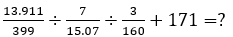106
175
150
125
205

Directions (11-15): In each of these questions, two equations (I) and (II) are given. You have to solve both the equations and give answer

Q11.if x > y
if x ≥ y
if x < y
if x ≤ y
if x = y or no relation can be established between ‘x’ and ‘y’.

Q12.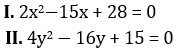if x > y
if x ≥ y
if x < y
if x ≤ y
if x = y or no relation can be established between ‘x’ and ‘y’.

Q13.if x > y
if x ≥ y
if x < y
if x ≤ y
if x = y or no relation can be established between ‘x’ and ‘y’.

Q14.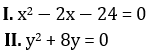if x > y
if x ≥ y
if x < y
if x ≤ y
if x = y or no relation can be established between ‘x’ and ‘y’.

Q15.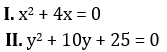if x > y
if x ≥ y
if x < y
if x ≤ y
if x = y or no relation can be established between ‘x’ and ‘y’.

You May also like to Read: